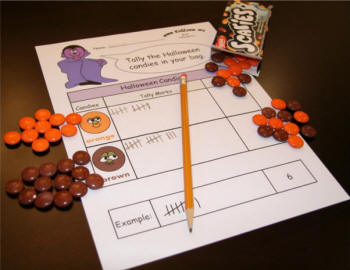#Halloween Themed Math Pages

Always remember that grade levels are not absolutes -- especially since we're all living in different countries and therefore have different curriculums.  Some kindergarten children will find the Grade One worksheets useful as will some Grade Two students.  Use your judgment!

NOTE:  Magic Squares definition:  add across and down to see the relationships in addition and subtraction.  Great self-checking review (the sums of the answers across = the sums of the answers down making it possible for the children to check their own answers)

1. Two numbers from 0 to 10

2. Fill in the blanks - two numbers from 0 to 10

3. Add a column of three numbers (the first number is always "1")

4. One number from 10 to 19 and one number from 0 to 9   (addition)   (subtraction)

5. Single digit number MAGIC SQUARES  (addition)   (subtraction)

6. Single digit number MATH TABLES  (addition)   (subtraction

7. Single digit number sentences - fill in the + or - sign (addition/subtraction

8. Halloween Graphing

9. Word Problems

1. One number from 10 to 99 and one number from 0 to 9   (addition)   (subtraction)

2. Number sentences - fill in the + or - sign (addition/subtraction

3. Two digit numbers - NO CARRYING   (addition)   (subtraction

4. Two digit numbers - CARRYING

5. Fill in the blanks - two digit numbers with CARRYING

6. Two digit number MAGIC SQUARES   (addition)   (subtraction

7. Two digit number MATH TABLES  (addition)   (subtraction

8. Two digit number sentences - fill in the + or - sign (addition/subtraction

9. Two numbers from 0 to 10   (multiplication)

10. Multiplication MATH TABLES  (multiplication)

11. Number sentences - fill in the + or - or x sign (addition/subtraction/multiplication

12. Halloween Graphing

13. Word Problems

1. Three digit number   (addition)   (subtraction

2. Fill in the blanks - three digit number   (addition)   (subtraction)

3. Three digit number MAGIC SQUARES   (addition)   (subtraction)

4. Three digit number MATH TABLES  (addition)   (subtraction

5. Four digit number   (addition)   (subtraction

6. Fill in the blanks - four digit number   (addition)   (subtraction)

7. Four digit number MAGIC SQUARES   (addition)   (subtraction)

8. Four digit number MATH TABLES  (addition)   (subtraction

9. Decimal numbers - NO CARRYING   (addition)   (subtraction)

10. Decimal numbers - CARRYING   (addition)   (subtraction)

11. Multiply one number from 10 to 100 and one number from 0 to 10

12. Find the missing factor (1 to 10)  (vertical)   (horizontal

13. Division - single digit quotient without remainders

14. Division - double digit quotient without remainders

15. Halloween Graphing

16. Word Problems

1. Five digit number   (addition)   (subtraction

2. Fill in the blanks - five digit number   (addition)   (subtraction)

3. Two digit decimal numbers   (addition)   (subtraction)

4. Multiply two digit numbers

5. Find the missing factor (1 to 100)  (vertical)   (horizontal

6. Two digit multiplication MATH TABLES  (multiplication)

7. Division - triple digit quotient without remainders

8. Division - Two digit division with remainders

9. Word Problems

1. Multiply three digit numbers

2. Three digit multiplication MATH TABLES  (multiplication)

3. Four digit multiplication MATH TABLES  (multiplication)

4. Multiply a decimal number by a whole number

5. Divide a decimal number by a whole number

6. Multiply decimal numbers

7. Word Problems

## Directions:

Click one of the links above.  Click once on the image and then print.  To generate a new worksheet, click the GENERATE NEW WORKSHEET link at the top right hand side of your page.